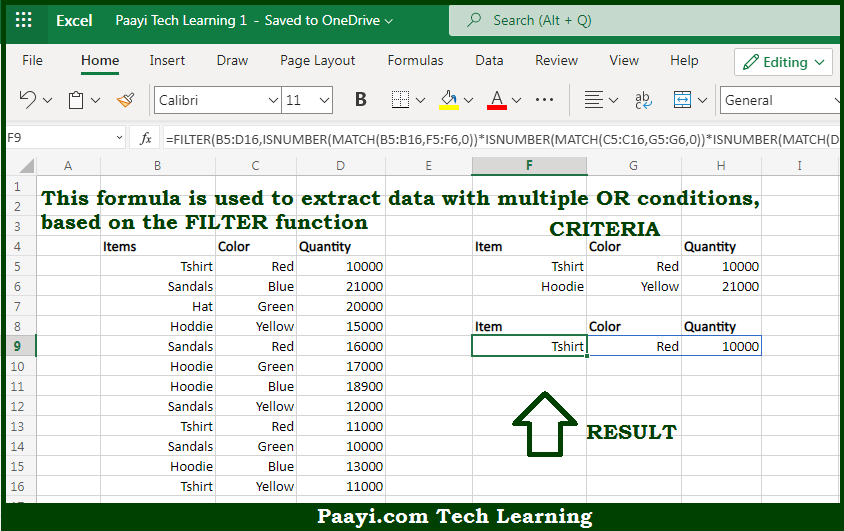# Learn How to Filter With Multiple OR Criteria in Microsoft Excel

Written by | 0 Comments | 497 Views

In this article, you will learn how to evaluate things in Dynamic Arrays with formulas in Microsoft Excel using a single/combination(s) of functions. You will also know How to Filter With Multiple OR Criteria and see the generic formula.

Learn How to Filter With Multiple OR Criteria in Microsoft Excel

The main purpose of this formula is to extract data with multiple OR conditions. Here we will learn how to filter with multiple OR criteria in the given data range in the workbook in Microsoft Excel. That implies, with the help of a formula based on the FILTER, and MATCH function you can able to extract data with multiple OR conditions. So, with the help of this formula, you can able to filter with multiple OR criteria in the given data range in the workbook in Microsoft Excel.

General Formula to Filter With Multiple OR Critera

=FILTER(B5:D16,ISNUMBER(MATCH(items,F5:F6,0))*ISNUMBER(MATCH(colors,G5:G6,0))*ISNUMBER(MATCH(cities,H5:H6,0)))

The Explanation for the Filter With Multiple OR CriteriaSo we know that with the help of the given formula above you can able to extract data with multiple OR conditions. Here we will learn how to filter with multiple OR criteria in the given data range in the workbook in Microsoft Excel. As we know that the filtering logic of this formula is the "include argument." Which is applied with the ISNUMBER and MATCH functions, together with boolean logic applied in an array operation. The MATCH function is configured "backward", with lookup values coming from the data. However, the criteria used for the lookup array. So, with the help of this formula, you can able to extract data with multiple OR conditions. Here we will learn how to filter with multiple OR criteria in the given data range in the workbook in Microsoft Excel.